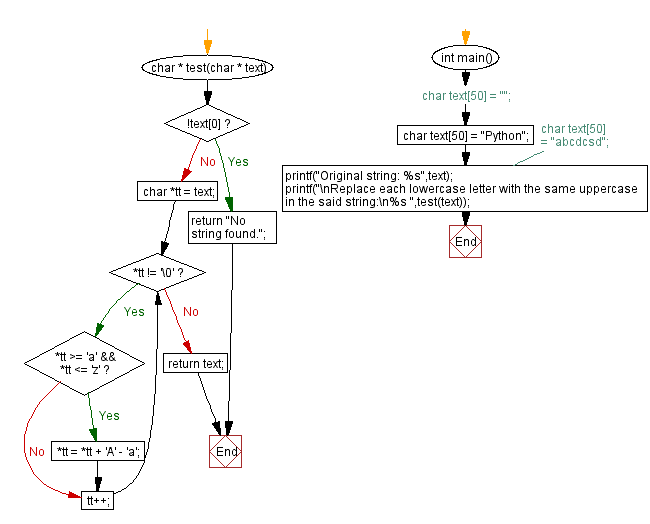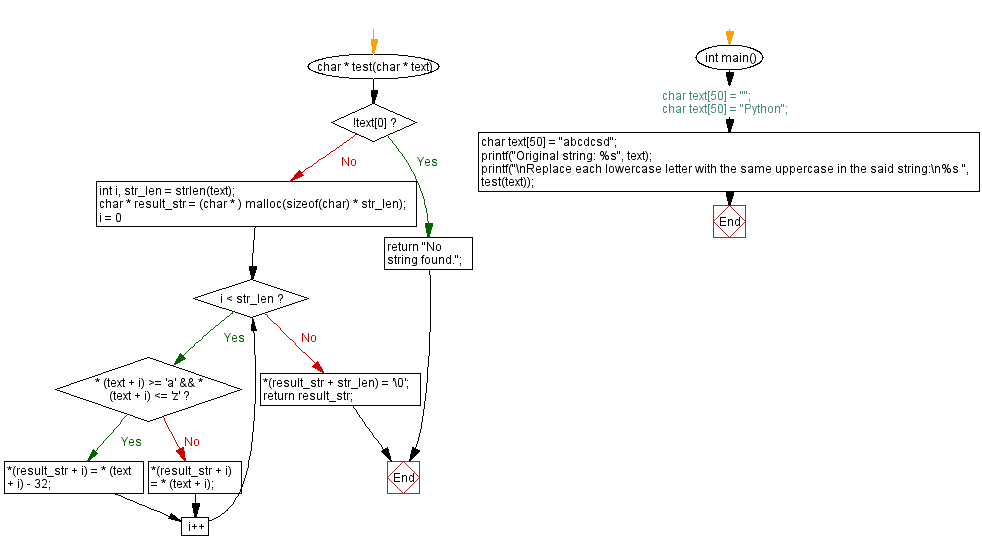﻿ C - Replace every lowercase letter with the same uppercase# C Programming: Replace every lowercase letter with the same uppercase

## C String: Exercise-40 with Solution

Write a C programming to replace each lowercase letter with the same uppercase letter of a given string. Return the new string.

Sample Data:

("Python") -> "PYTHON"
("abcdcsd") -> "ABCDCSD"

Sample Solution-1:

C Code:

``````#include <stdio.h>
#include <string.h>
#include <stdlib.h>
char * test(char * text) {
if (!text)
return "No string found.";
char *tt = text;
while(*tt != '\0')
{
if (*tt >= 'a' && *tt  <= 'z')
{
*tt = *tt + 'A' - 'a';
}
tt++;
}
return text;
}
int main() {
//char text = "";
char text = "Python";
//char text = "abcdcsd";
printf("Original string: %s",text);
printf("\nReplace each lowercase letter with the same uppercase in the said string:\n%s ",test(text));
return 0;
}
```
```

Sample Output:

```Original string: Python
Replace each lowercase letter with the same uppercase in the said string:
PYTHON
```

Flowchart :Sample Solution-2:

C Code:

``````#include <stdio.h>
#include <string.h>
#include <stdlib.h>

char * test(char * text) {
if (!text)
return "No string found.";
int i, str_len = strlen(text);
char * result_str = (char * ) malloc(sizeof(char) * str_len);
for (i = 0; i < str_len; i++) {
if ( * (text + i) >= 'a' && * (text + i) <= 'z') {
*(result_str + i) = * (text + i) - 32;
} else {
*(result_str + i) = * (text + i);
}
}

*(result_str + str_len) = '\0';
return result_str;
}

int main() {
//char text = "";
//char text = "Python";
char text = "abcdcsd";
printf("Original string: %s", text);
printf("\nReplace each lowercase letter with the same uppercase in the said string:\n%s ", test(text));
}
```
```

Sample Output:

```Original string: abcdcsd
Replace each lowercase letter with the same uppercase in the said string:
ABCDCSD
```

Flowchart :C Programming Code Editor:

Improve this sample solution and post your code through Disqus.

Previous C Programming Exercise: Longest Palindromic Substring from a given string.
Next C Programming Exercise: Length of longest common subsequence of two strings

What is the difficulty level of this exercise?

Test your Programming skills with w3resource's quiz.

﻿